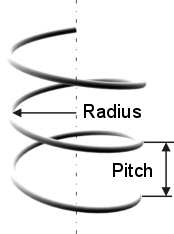# Calculating the Evolute of a Helix

## Calculating the Length of a Helical Shape

This page will allow you to calculate the evolute of a circular helix, also known as a cylindrical helix.

You will also get the constant angle which makes up the evolute with its plane perpendicular to the helix’s axis.

Some reasons you may want to know this would be to find the length of a handrail or a pitch line when building a spiral staircase, finding the length of the iron of a corkscrew, determining the linear speed at the end of a moving propeller or the evolute length of a screw’s thread, the length of wire in a spring, the length of an helical shape and so on.

## Calculating an Evolute Helix:

 Radius: Helix pitch: Unit: mm cm dm m inch foot yard Angle of rotation*: ° Decimal places: 0 1 2 3 4 5 6 7 8 9 10
 Height of the Helix: 2,80 m Circumference (of one loop): 5,03 m Length of a helix’s evolute: 5,75 m Constant angle*: 29,12 °* The angle of rotation is measured in degrees °. If the helix loops multiple times, don't forget to multiply the number by 360 for each loop.

* The constant angle corresponds to the angle formed from the tangent of the spiral’s wire and from the plane orthogonal to the axis of the cylinder. More simply, when the spiral is placed vertically, such as the drawing above, this is the angle formed between the horizontal plane and the spiral’s wire.